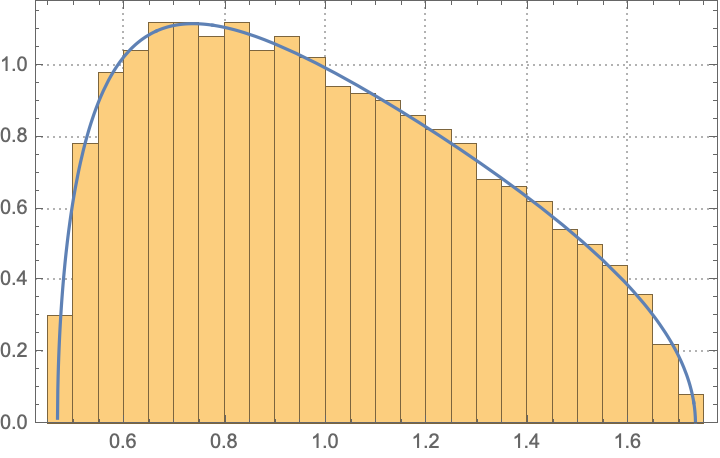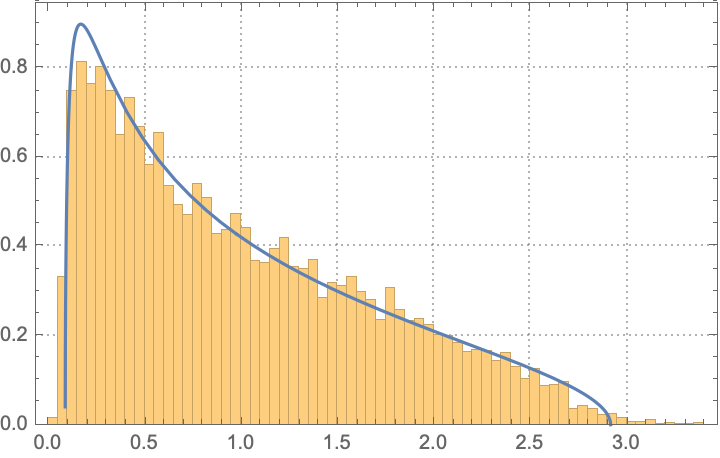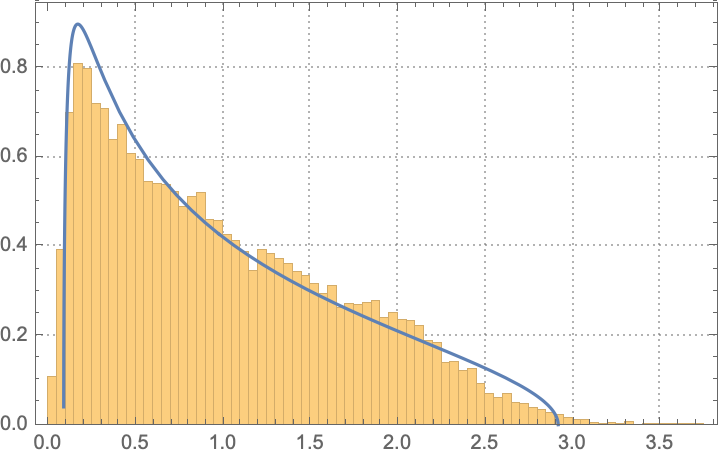# Singular values of random uniform matrix

Suppose $$X \in \mathbb{R}^{N \times M}$$ with elements sampled i.i.d. from $$\mathcal{U}(-\sigma, \sigma)$$.

I would like to find the marginal distribution of the unordered singular values of $$X$$. The case for $$X \sim \mathcal{N}(0, \sigma)$$ has been extensively studied, but i can't seem to find any work on the uniform distribution case.

Or equivalently the distribution of the eigenvalues of $$X^TX$$. The distribution of the $$X^TX$$ seems to follow some gaussian with mean zero and a small gaussian in the tail as observed in some simulations. This leads to the eigenvalues not being distributed as gaussian i.i.d.Rescale $$Y=(\sqrt{3}/\sigma)X$$, so that the matrix elements of $$Y$$ have uniform distribution with zero mean and unit variance.

In the limit $$N,M\rightarrow\infty$$ at fixed $$M/N=r\in(0,1]$$ the $$M$$ eigenvalues $$\lambda_n$$ of $$N^{-1}Y^{\rm T}Y$$ have the Marcenko-Pastur distribution, $$\rho(\lambda)=\frac{1}{2\pi \lambda r}\sqrt{(\lambda_+-\lambda)(\lambda-\lambda_-)},\;\;\lambda_-<\lambda<\lambda_+,\;\;\lambda_\pm=(1\pm\sqrt r)^2.$$ For a proof, see for example these notes.

The plot below is a test for $$M=10^3$$, $$N=10^4$$: the histogram shows the eigenvalue distribution for a randomly generated matrix with uniformly i.i.d. matrix elements, the curve is the Marcenko-Pastur distribution, which as you can see agrees very nicely.
(No idea why the histogram in the OP is so different.)The OP has asked in the comment for non-asymptotic results. I don't think there are exact closed-form expressions for any $$N,M$$, but the large-$$N$$,$$M$$ asymptotics is already reached quite accurately for moderately large values of $$N,M$$. In the plot below I show the case $$M=10,N=20$$, which is already quite close to the Marcenko-Pastur limit.And even $$M=5,N=10$$ is not too bad...• Thanks for the quick reply. I was incomplete in my question. I am aware of the MP distribution, but i am looking for a general pdf or marginal distribution and not the limiting case since the applications requires all sizes of matrices. – David Vander Mijnsbrugge Apr 9 at 20:21
• you are looking for an exact result for any $M,N$ ? that will not be forthcoming, I'm afraid; however, I'm pretty confident that the "large-$M,N$" limit is reached with good accuracy already for moderately large values of $M,N$, say upwards of 10 or so. – Carlo Beenakker Apr 9 at 20:26
• I have added two plots to illustrate this. – Carlo Beenakker Apr 9 at 20:58
• Thank you, it seems that i have some accuracy issues with my code. Thank you for the clarification. – David Vander Mijnsbrugge Apr 10 at 7:34